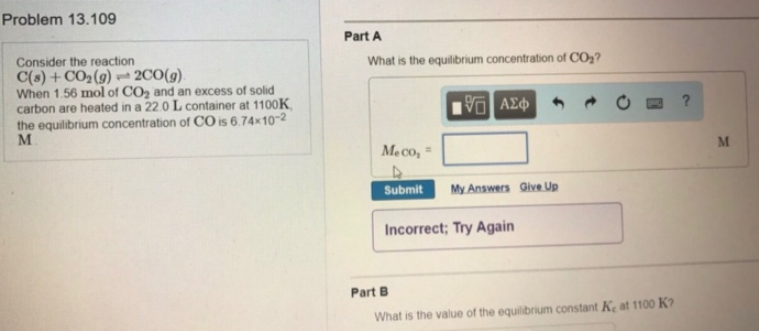# Problem: Consider the reaction C(s) + CO2(g) ⇌ 2CO(g). When 1.56 mol of CO2 and an excess of solid carbon are heated in a 22.0 L container at 1100K, the equilibrium concentration of CO is 6.74 x 10-2 M. What is the equilibrium concentration of CO2? What is the value of the equilibrium constant Kc at 1100 K?

###### FREE Expert Solution
83% (404 ratings)###### Problem Details

Consider the reaction

C(s) + CO2(g) ⇌ 2CO(g).

When 1.56 mol of CO2 and an excess of solid carbon are heated in a 22.0 L container at 1100K, the equilibrium concentration of CO is 6.74 x 10-2 M.

What is the equilibrium concentration of CO2

What is the value of the equilibrium constant Kc at 1100 K?